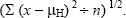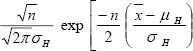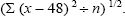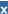U.S. Department of Transportation
1200 New Jersey Avenue, SE
Washington, DC 20590
202-366-4000

Federal Highway Administration Research and Technology
Coordinating, Developing, and Delivering Highway Transportation InnovationsThis report is an archived publication and may contain dated technical, contact, and link information
 Federal Highway Administration > Publications > Research Publications > LTPP Publications > 03093 > Appendm.Cfm Study of Long-Term Pavement Performance (LTPP): Pavement Deflections
Publication Number: FHWA-RD-03-093
Date: August 2006

# Study of Long-Term Pavement Performance (LTPP): Pavement Deflections

## Appendix M. The Generalized Likelihood Ratio For H48 Over H60

If two hypotheses are available for a model for data, a standard way to compare them is the generalized likelihood ratio. Briefly, this is a computation of the highest possible probability for the data under each hypothesis, or the maximum likelihood estimates of the parameters for the data within each hypothesis. The H48 hypothesis is that sensor 7 was offset 121.9 cm (48 inches); the H60 hypothesis is that sensor 7 was offset 152.4 cm (60 inches).

In each of the three cases examined below, the data to be modeled are the predicted positions of sensor 7, using all of the nonjoint associated deflection data for a particular site and day. These data fail tests of the hypothesis that they come from a normal distribution, but are approximately symmetric. The central limit theorem would then imply that the sample means have approximate normal distributions, with the approximation improving as the sample size (i.e., the number of dates) increases.

Thus the sample mean, x bar, is approximately normally distributed with mean µH and unknown standard deviation s divided by the square root of n . Here µH is the appropriate value dictated by the hypothesis; if H is H48, then µH is 48, and if H is H60, then µH is 60. The nuisance parameter s = sH is estimated by its maximum likelihood estimate shown in the equation given in figure 57.Figure 57. Equation. Maximum likelihood estimate.

The likelihood under either hypothesis is shown in the equation in figure 58.Figure 58. Equation. The likelihood.

For example, if the hypothesis is H48, then the value of µH is replaced by 48, and the value of sH is replaced as shown in the equation in figure 59.Figure 59. Equation. Maximum likelihood estimate, sH = 48.

This likelihood value is computed for each hypothesis, and the ratio of these two numbers is called the likelihood ratio, a computation of the relative likelihood of the data under the two competing hypotheses.

Table 12 captures all of the relevant information for the three datasets presented in the report. MLE of SD is the maximum likelihood estimate of s, the standard deviation, referenced above.

Table 12. Likelihood ratio stats for protocol versus nonprotocol d7 sensor positions.
FWD Serial Number Sample Mean,MLE of SD under H48 MLE of SD under H60 Sample Size, n Likelihood under H48 Likelihood under H60 Likelihood Ratio
8002–129 47.99 1.88 12.16 12 0.7350095 0.0003252 2,260
8002–132 48.12 2.33 12.10 29 0.8840417 1.531E–07 5,773,093
8002–061 47.20 1.44 12.87 65 9.4002E–05 2.789E–15 33,706,837,035